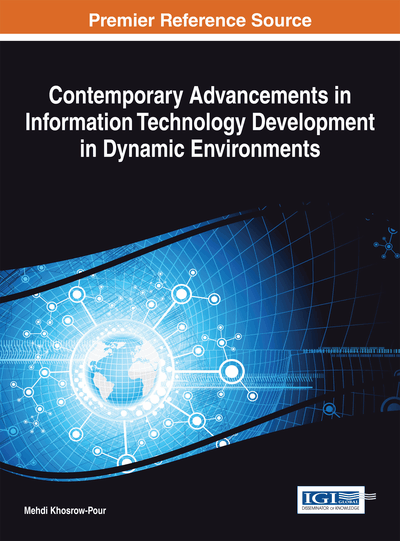# A Generalized Graph Strict Strong Coloring Algorithm: Application on Graph Distribution

Meriem Bensouyad (University Constantine 2, Algeria), Mourad Bouzenada (University Constantine 2, Algeria), Nousseiba Guidoum (University Constantine 2, Algeria) and Djamel-Eddine Saïdouni (University Constantine 2, Algeria)
Copyright: © 2014 |Pages: 20
DOI: 10.4018/978-1-4666-6252-0.ch010

## Abstract

This chapter examines the graph coloring problem. A graph strict strong coloring algorithm has been proposed for trees in Haddad and Kheddouci (2009). In this chapter, the authors recall the heuristic-based algorithm for general graphs named GGSSCA (for Generalized Graph Strict Strong Coloring Algorithm) proposed in Bouzenada, Bensouyad, Guidoum, Reghioua, and Saidouni (2012). The complexity of this algorithm is polynomial with considering the number of vertices. Later, in Guidoum, Bensouyad, and Saidouni (2013), GGSSCA was applied to solve the graph distribution problem.
Chapter Preview
Top

## 1. Introduction

The graph coloring is a combinatorial optimization problem. The coloring process is done according to some properties. Let G be a given graph, a proper vertex coloring of a graph is a vertex coloring such that no two adjacent vertices have the same color. The minimum number of colors among all proper colorings of G is its chromatic number χ(G). It is well known that the k-coloring problem for general graphs is NP-complete and the calculation of the chromatic number is NP-hard (Klotz, 2002).

A plenty variety of graph coloring is presented in Chen, Gyarfas, and Schelp (1998). Some others colorings deal with edges of graphs (Caragiannisa, Kaklamanisa, & Persianob, 2002), others with lists (Albertson, Grossman, & Haas, 2000). In addition too, solutions for coloring both vertices and edges of graphs have been proposed (Borodin, Kostochka, & Woodall, 1998). Often, graph coloring and dominance problems are correlated. Several relations between the chromatic number and some dominance parameters are presented in Chellali and Volkmann (2004).

In Zverovich (2006), a notion of graph strong coloring has been introduced. It defines a dominance relation between graph vertices and color classes. Strong coloring problem has been shown as NP-complete. After that, in Haddad and Kheddouci (2009), authors defined a strict strong k-coloring (k-SSColoring) of graphs, which is a strong k-coloring with a non-empty constraint on color classes (Figure 1). The new coloring parameters define the dominance property.

An exact polynomial time of graph strict strong coloring algorithm for trees has been proposed.

The Graph Strict Strong Coloring Problem (GSSCP) consists to find the minimum number of colors which check the dominance property for each vertex. In fact, finding exact solutions for general graphs are NP-complete (Haddad & Kheddouci, 2009).

As an application of such coloring process, we can cite broadcasting (Haddad, Dekar, & Kheddouci, 2008), data dissemination, gossiping and graph distribution (Guidoum et al., 2013). This latter is introduced to deal with state space explosion problem which is a fundamental obstacle in formal verification of concurrent systems. The graph distribution problem will be detailed later.

In this chapter, we illustrate that heuristic based algorithms may be a good approach to solve graph strict strong coloring problem for general graphs. Especially, we focus on the GGSSCA algorithm (for Generalized Graph Strict Strong Coloring Algorithm) which has a polynomial complexity with respect to the number of vertices (Bouzenada et al., 2012).

In the other hand, we also show that coloring concept can be useful to solve the graph distribution problem by means of the SSCGDA algorithm (for Strict Strong Coloring based-Graph Distribution Algorithm) proposed in Guidoum et al., (2013).

The chapter is organized as follows: Section 2 gives some preliminary notations and definitions related to graph coloring. In Section 3, the GGSSCA is described. Section 4 presents GGSSCA’s experimental results. In section 5, GGSSCA is applied on trees and results are compared with those of the exact algorithm.

In section 6, GGSSCA is adapted to solve the graph distribution problem. Specifically, in this section, the graph distribution problem is introduced then the relation between graph distribution and graph strict strong coloring is shown. Next, the GGSSCA application named SSCGDA (for Strict Strong Coloring based Graph Distribution Algorithm) is illustrated. Finally, various experimental results related to SSCGDA are presented.

Top

## 2. Preliminary Defenitions

In this chapter, we mean by graph a simple undirected loopless graph with no isolated vertices.

Let G = (V, E) be a graph such that V = {v1, v2... vn} is a finite set of vertices and EV × V is a finite set of edges.

## Complete Chapter List

Search this Book:
Reset